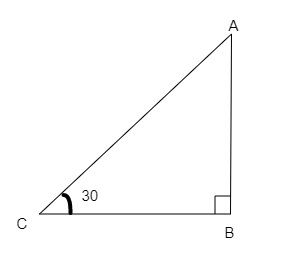# A circus artist is climbing a $20 \mathrm{~m}$ long rope, which is tightly stretched and tied from the top of a vertical pole to the ground. Find the height of the pole, if the angle made by the rope with the ground level is $30^{\circ}$.

Given:

Length of the rope$=20\ m$.

The angle made by the rope with the ground level is $30^{\circ}$.

To do:

We have to find the height of the pole.

Solution:Let AB be the height of the pole and AC the length of the rope.

Angle made by the rope with the ground $\angle BCA=30^o$

Therefore,

$sin30^o=\frac{AB}{AC}$

$\frac{1}{2}=\frac{AB}{20}$

$AB=\frac{20}{2}$

$AB=10\ m$

The height of the pole is $10\ m$.

Updated on: 10-Oct-2022

70 Views